Finding Inverse

Chapter 1 Class 12 Relation and Functions
Concept wise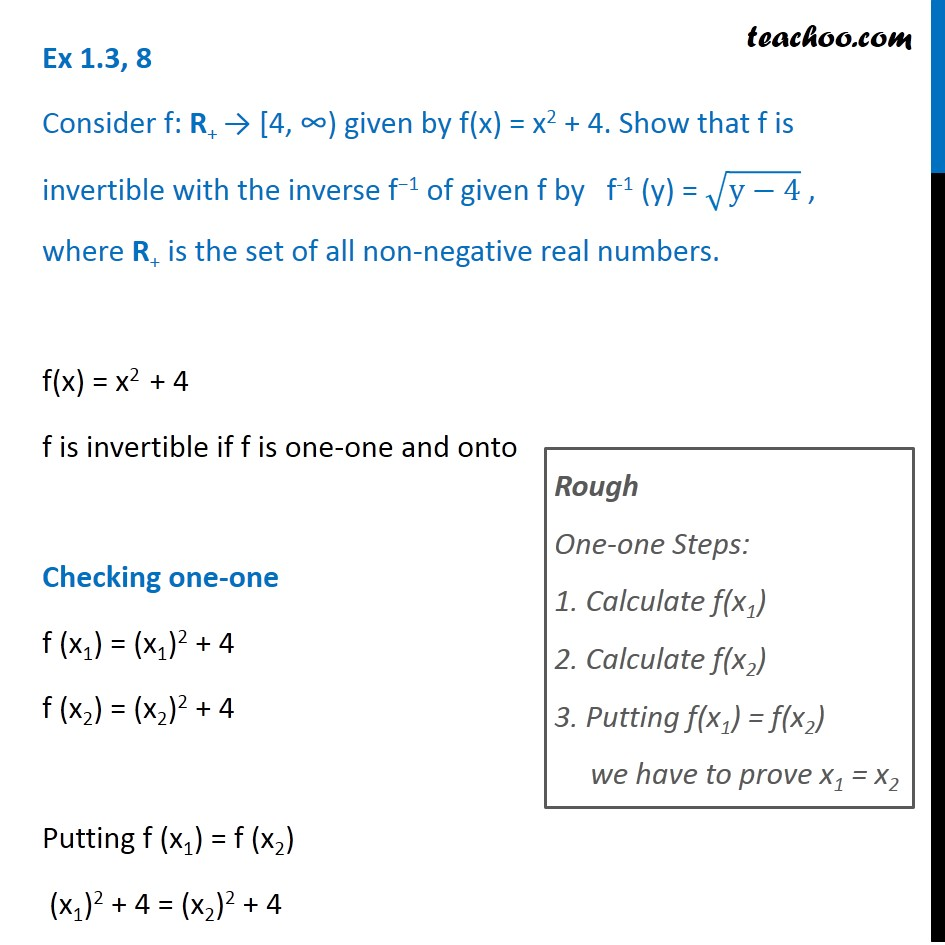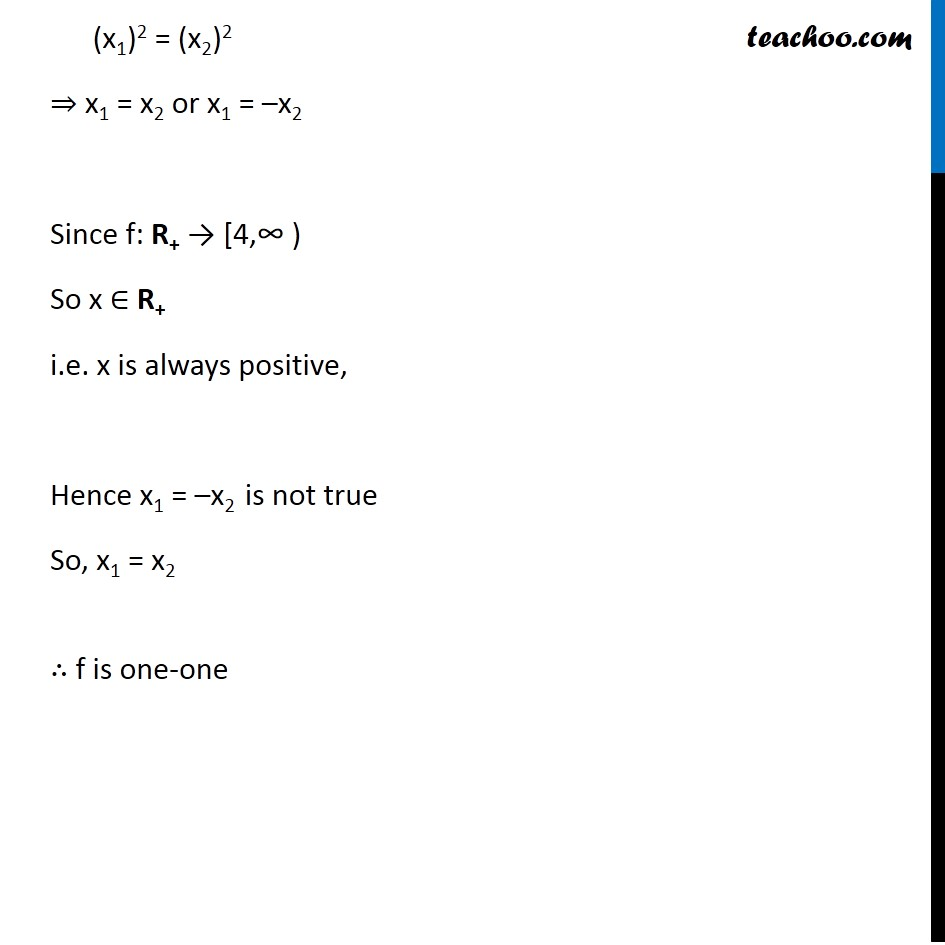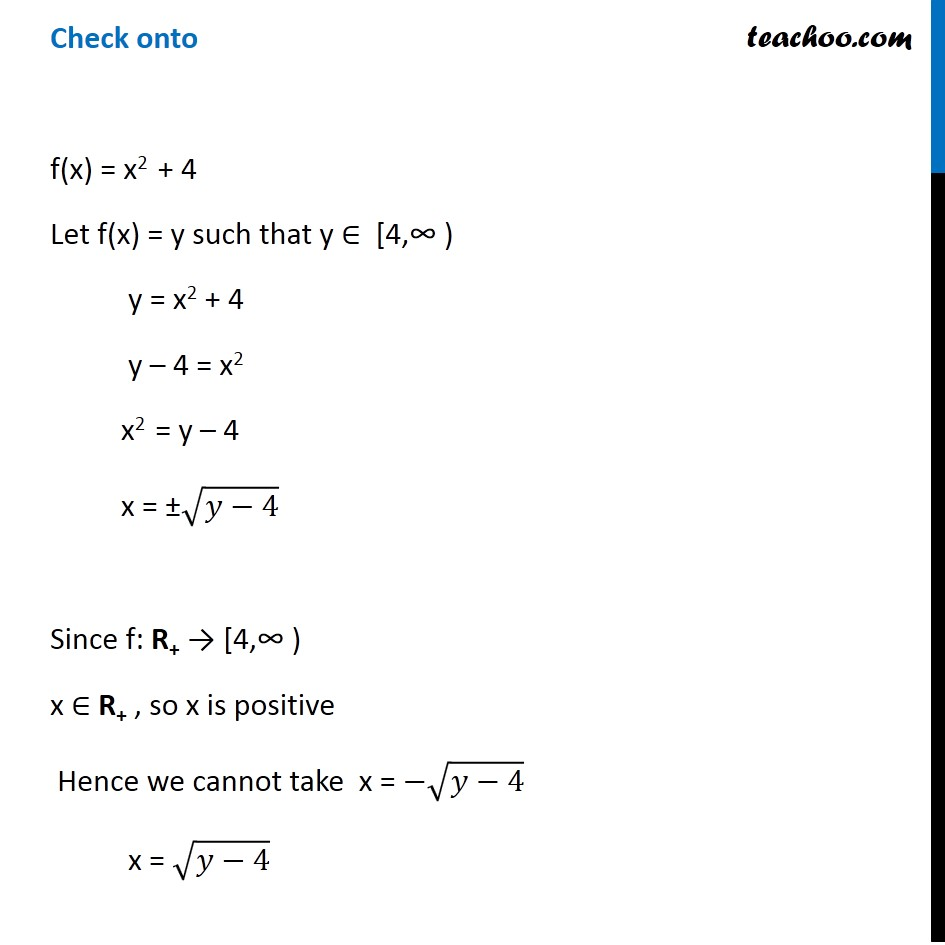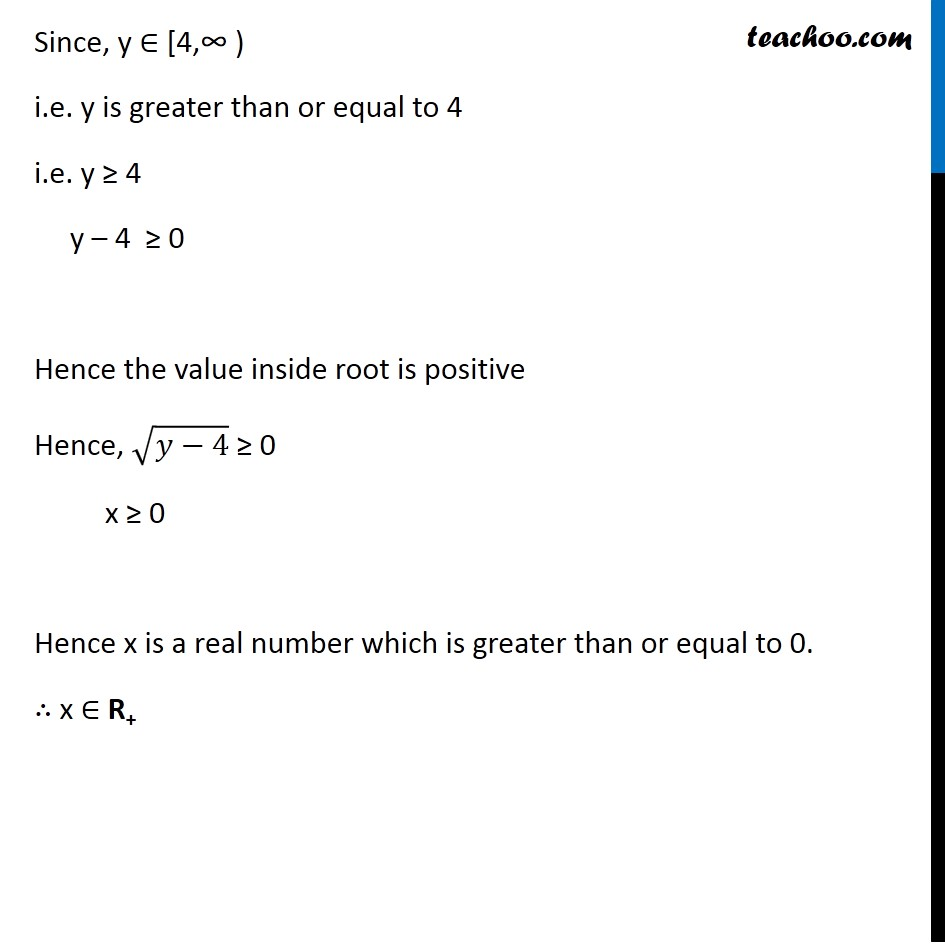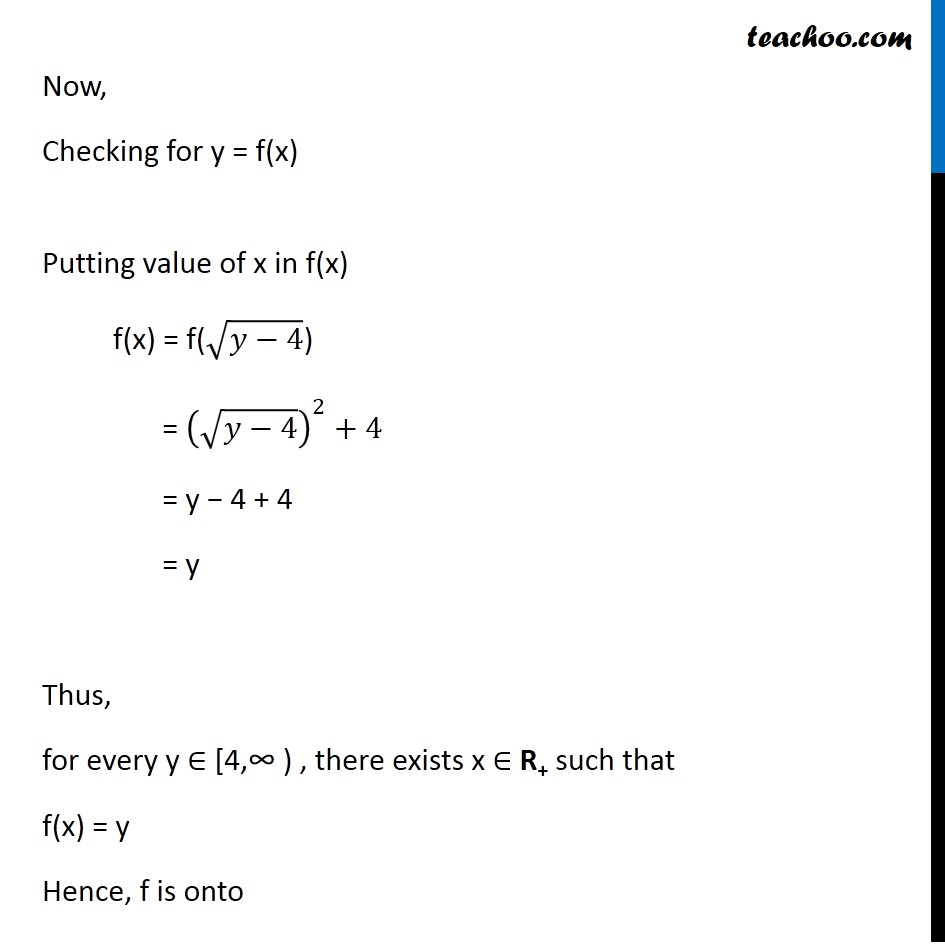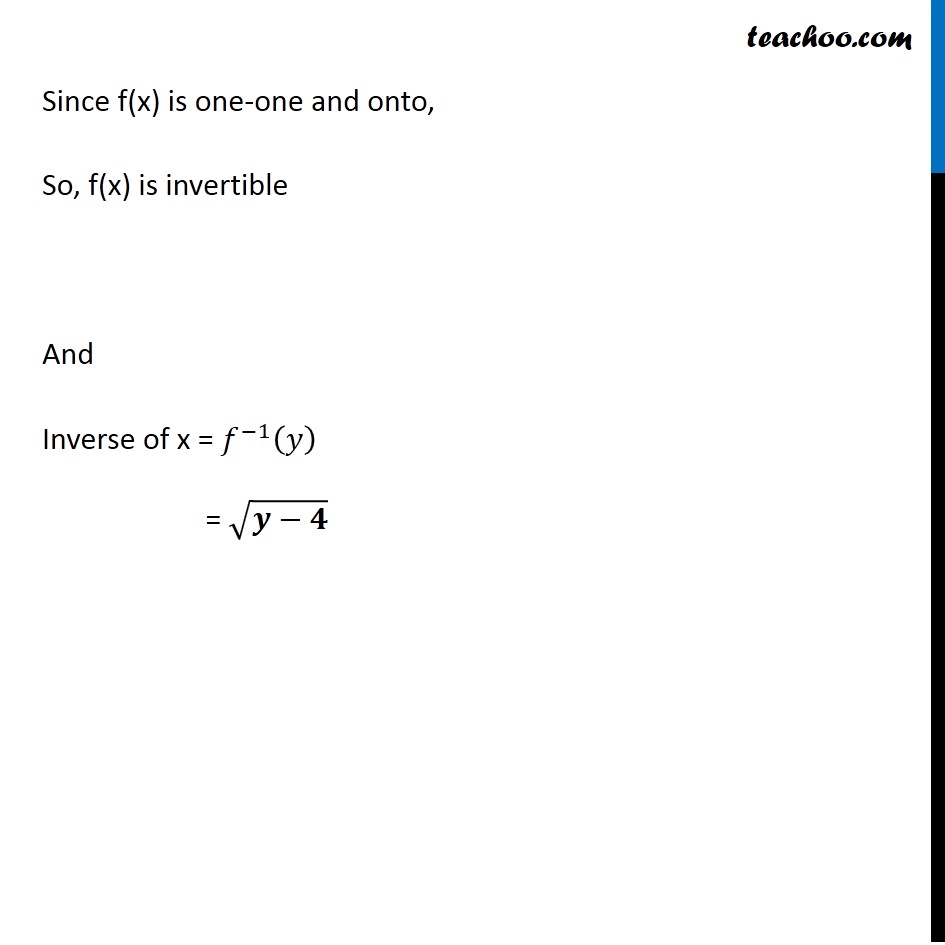Introducing your new favourite teacher - Teachoo Black, at only ₹83 per month

### Transcript

Ex 1.3, 8 Consider f: R+ → [4, ∞) given by f(x) = x2 + 4. Show that f is invertible with the inverse f−1 of given f by f-1 (y) = √(y−4) , where R+ is the set of all non-negative real numbers. f(x) = x2 + 4 f is invertible if f is one-one and onto Checking one-one f (x1) = (x1)2 + 4 f (x2) = (x2)2 + 4 Putting f (x1) = f (x2) (x1)2 + 4 = (x2)2 + 4 Rough One-one Steps: 1. Calculate f(x1) 2. Calculate f(x2) 3. Putting f(x1) = f(x2) we have to prove x1 = x2 (x1)2 = (x2)2 ⇒ x1 = x2 or x1 = –x2 Since f: R+ → [4,∞ ) So x ∈ R+ i.e. x is always positive, Hence x1 = –x2 is not true So, x1 = x2 ∴ f is one-one Check onto f(x) = x2 + 4 Let f(x) = y such that y ∈ [4,∞ ) y = x2 + 4 y – 4 = x2 x2 = y – 4 x = ±√(𝑦−4) Since f: R+ → [4,∞ ) x ∈ R+ , so x is positive Hence we cannot take x = −√(𝑦−4) x = √(𝑦−4) Since, y ∈ [4,∞ ) i.e. y is greater than or equal to 4 i.e. y ≥ 4 y – 4 ≥ 0 Hence the value inside root is positive Hence, √(𝑦−4) ≥ 0 x ≥ 0 Hence x is a real number which is greater than or equal to 0. ∴ x ∈ R+ Now, Checking for y = f(x) Putting value of x in f(x) f(x) = f(√(𝑦−4)) = (√(𝑦−4))^2+4 = y − 4 + 4 = y Thus, for every y ∈ [4,∞ ) , there exists x ∈ R+ such that f(x) = y Hence, f is onto Since f(x) is one-one and onto, So, f(x) is invertible And Inverse of x = 𝑓^(−1) (𝑦) = √(𝒚−𝟒)